# Introducing the Sedenion Fano Tesseract Mnemonic

The Sedenion “Fano Tesseract” Mnemonic is an extension of the “Fano Cube” idea introduced by John Baez in his much cited blog post. The Fano Cube identifies each valid each triad by a hyper-plane which intersects the e_0 node.

Notice in this VisibLie_E8 output for the pane #3 “Fano Visualization Demonstration”, there are 35 sedenion triads, 7 of which are from the octonion used as an upper left quadrant base for a Cayley-Dickson doubling (highlighted in red).

The 16 vertices of the tesseract are sorted by the same “triad flattening” process used to construct a consistent Fano Plane Mnemonic for all 480 unique octonion multiplication tables.

As in the Fano Cube, the edges are highlighted in Cyan if they are selected in the n1-n3 buttons. Unlike the Fano Plane and Cube, the edges represented by the split octonion multiplication table columns/rows are not highlighted in red.

While there are 32 edges in the formal tesseract, each valid sedenion triad is identified by a hyper-plane which intersects the e_0 node, which are not necessarily those of the formal tesseract.

Here is the computation of the same sedenion table given in the Sedenion Wikipedia article as well as from this website: http://www.derivativesinvesting.net/article/307057068/a-few-hypercomplex-numbers/, which uses the harder to read IJKL style notation.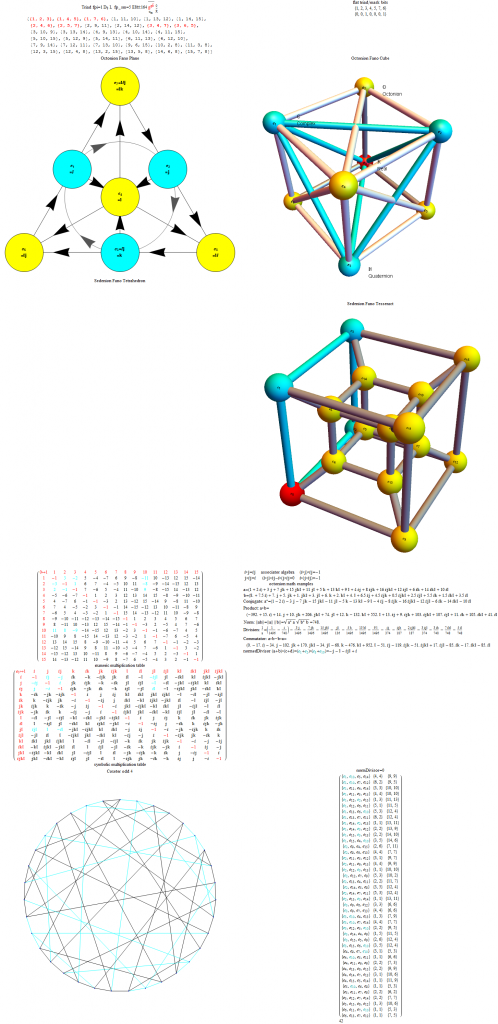# Incorporating Sedenions into the VisibLie_E8 visualizer

It is taken from an example given on this website: http://www.derivativesinvesting.net/article/307057068/a-few-hypercomplex-numbers/, which uses the harder to read IJKL style notation.

Compare the upper left quadrant of the multiplication table. It is the same as the octonion of E8 #164 (non-Flipped) used to generate the sedenion using the Cayley-Dickson doubling. It can be read off using the Fano Plane (or Fano Cube) mnemonic. My next post will show the Sedenion forms of these, namely the Fano Tetrahedron and Tesseract!

Notice the highlighting of the interesting aspect of sedenions, where some non-zero p=a+b and q=c+d give a product pq=0. These are given in a list of the {a,b,c,d} where p={a+b} and q={c+d} and pq=0 (along with the deltas between the indices), always 42 excluding trivial juxtapositions. In this case, it is the row entry with {3,12,5,10}.

Here is the computation of the same table in the format given in the Sedenion Wikipedia article.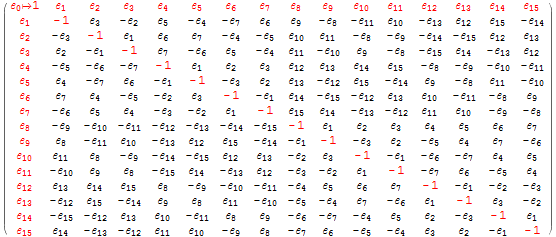# G2 as the automorphism group of the (split) octonion algebra

This post describes the derivation of G2 automorphisms for each of the 480 unique octonion multiplication tables, as well as each of the 7 split octonions (created from negating the 4 row/column entries which are not members of the split #, which is an index to one of the 7 triads that make up the octonion).

The Exceptional Lie Algebra/Group G2 is identified by its Dynkin diagram and/or associated Cartan Matrix (also shown here with its Hasse diagram):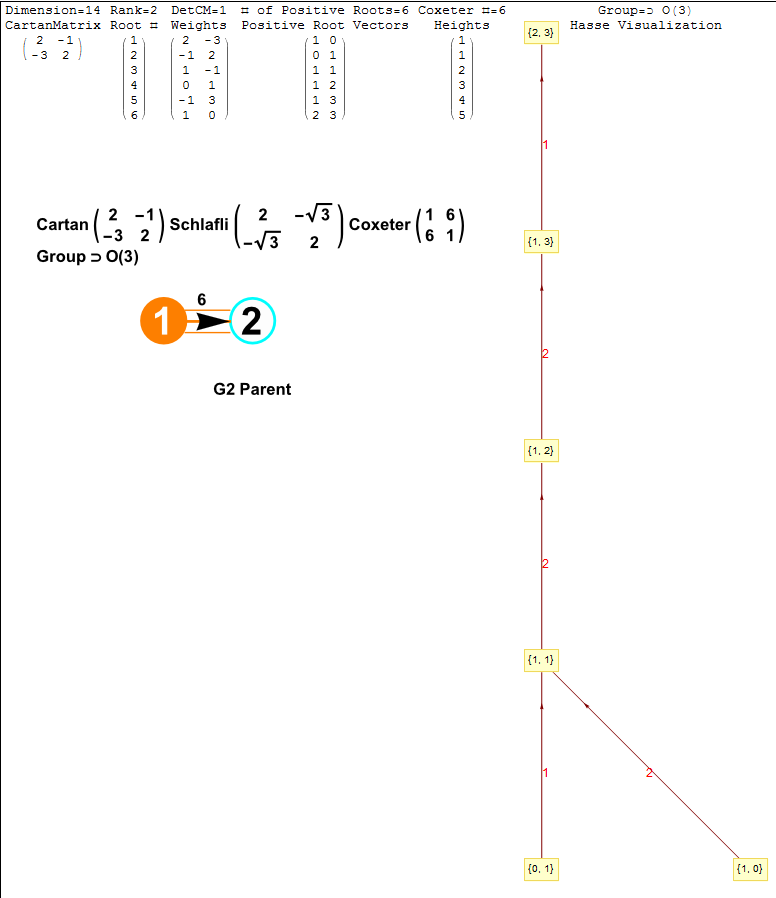The particular octonion multiplication table selected in the example referenced above is associated in my model as a “Non Flipped E8 #164”. The multiplication table in various formats (IJKL, e_n, and Numeric) is: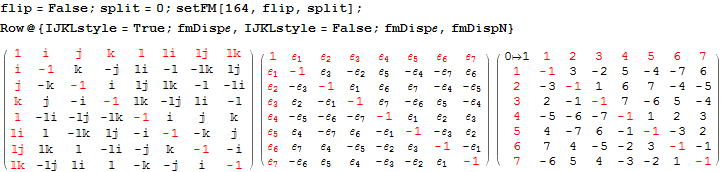The selected multiplication table (fm) is the basis for the following octonion symbolic math:
[wlcode](* Octonion math from the matrix using 8D vector representations for x and y, this has the Subscript[\[ScriptE], 0]=1 first *)
octProduct[a_, b_] := Block[{c3 = Array[0 &, 8]},
Do[c3[[If[i != j, Abs@fm[[i, j]] + 1, 1]]]+=Sign@fm[[i, j]] a[[i]] b[[j]],
{i, 8}, {j, 8}];
Chop@c3];

(* Complex, quaternion and octonian multiplication Subscript[\[ScriptE], a]\[SmallCircle]Subscript[\[ScriptE], b] *)
quat2oct@in_ := Block[{a3},
If[QuaternionQ@in,
a3 = FromQuaternion@in;
Switch[Length@a3,
0, Re@a3 + Im@a3 Subscript[\[ScriptE], 1],
2, If[Length@a[] > 0, a3,
1\[SmallCircle](Re@a3[] +
Im@a3[] Subscript[\[ScriptE], 1]) + a3[]]],
3, Re@a3[] + Im@a3[] Subscript[\[ScriptE], 1] +
a3[[2 ;;]]],
“not Quaternion”] /. Most@Thread[octIJKL -> oct]];

(* Octonion math with conversions, leave final as octonion *)
SmallCircle[a_List, b_List] := octonion@octProduct[a, b];
SmallCircle[a_, b_Quaternion] := a\[SmallCircle]quat2oct@b;
SmallCircle[a_Quaternion, b_] := quat2oct@a\[SmallCircle]b;
SmallCircle[a_, b_Complex] := a\[SmallCircle]ToQuaternion@b;
SmallCircle[a_Complex, b_] := ToQuaternion@a\[SmallCircle]b;
SmallCircle[a_, b_] := oct2List@a\[SmallCircle]oct2List@b;

associator[a_List, b_List,c_List] := (a\[SmallCircle]b)\[SmallCircle]c-a\[SmallCircle](b\[SmallCircle]c);
associator[a_, b_, c_] :=associator[oct2List@a, oct2List@b, oct2List@c];[/wlcode]

Each set of 21 upper triangle pairs of octonion elements (utOct) has 14 “derivations” which are null
(alternatively, the NullSpace of the derivation vectors of utOct has 7 which are NOT null…)

A derivation pair D_{x, y} is defined by:
[wlcode](* Commutator *)
commutator[x_,y_]:=x\[SmallCircle]y-y\[SmallCircle]x;
CircleDot[x_,y_]:=commutator[x, y];

(* Commutator matrix *)
comMat[x_, y_] := x.y – y.x;
comMat[{x_, y_}] := comMat[x, y];

(* Derivation matrix and vector *)
derivation[x_,y_][a_]:=(x\[CircleDot]y)\[CircleDot]a-3 associator[x,y,a];
Square[{x_, y_, a_}] := derivation[x, y][a];
derMatrix[x_,y_]:=Table[Coefficient[\[Square]{x,y,oct[[i]]},oct[[j]]], {j, 7}, {i,7}];
derVector[x_,y_]:=derMatrix[x, y]//Flatten;[/wlcode]
The 14 null pairs define G2 for a SPECIFIC octonion multiplication matrix.

For each of 7 non-null derivations there is a triple of D_{x1, y1}=D_{x2, y2}+D_{x3, y3} related derivations.

There is a 7×21 matrix describing the non-NullSpace for the octonion given in the Wolfram MathSource reference above along with the 7 non-null triples. This is generated by g2Null:
[wlcode]g2Null:=NullSpace[derVector[Subscript[\[ScriptE], #1], Subscript[\[ScriptE],#2]]&@@@utOct//Transpose];

(* Formatting all non-Null upper triangle pairs derivation entries *)
outG2Null := MatrixForm[parallelMap[Grid[{
{Row[
Subscript[D, Row@Flatten@##] & /@
Partition[Flatten@{utOct[[Flatten@Position[#, -1]]]}, 2]]},{“==”},
{Row[
Subscript[D, Row@Flatten@##] & /@
Partition[Flatten@{utOct[[Flatten@Position[#, 1]]]}, 2]]}(*),
{“\[LongDash]\[LongDash]\[LongDash]\[LongDash]”}**)}] &,
g2Null]];[/wlcode]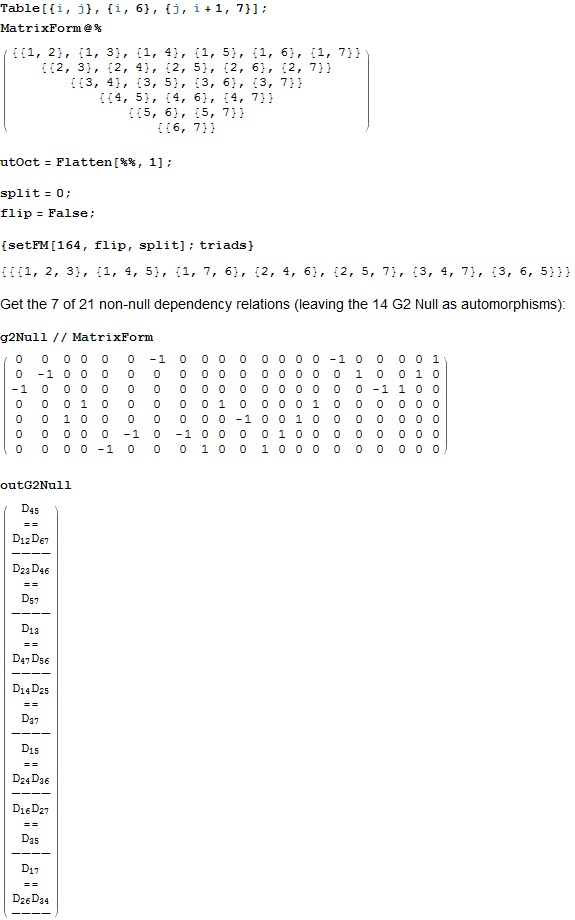getG2Null (below) retrieves two octonion multiplication matrices for the flipped and non-flipped index on one of 240 E8 particles.
[wlcode]formG2Null := MatrixForm@{
Row@{Style[Row@{“flip=”, flip}, Blue],
Style[” split # \[DownArrow],”, Red]},
g2NullTbl = Table[{
split,
setFM[#, flip, split];
outG2Null},
{split, 0, 7}];
MatrixForm[Column[#, Center] & /@ noNull@# & /@ Table[
(* Color the common Der in each column *)
cmnD =first@Select[g2NullTbl[[All,2,1,i,1,3,1,1,All]],
Length@# == 1 &];
cmnDclr = Style[cmnD, Magenta];
If[j == 1, {“”, Row@{
(* Highlight the missing index in the triple D_{x,y} for each row *)
Style[Complement[Range@7,Union@Flatten@g2NullTbl[[All,2,1,i,1,3,1,1,All,2,1]]][],Darker@Green],
g2NullTbl[[1, 2, 1, i]] /. cmnD -> cmnDclr}},
(* Display only non-null derivations from the splits if not the same as the base octonion *)
If[g2NullTbl[[1,2,1,i]] =!= g2NullTbl[[j,2,1,i]],
{Style[g2NullTbl[[j, 1]], Red],
g2NullTbl[[j,2,1,i]] /. cmnD -> cmnDclr}]],
{i,7},{j,8}]]} &;

getG2Null := ColumnForm[{
Style[Row@{“E8#=”, #}, Darker@Green],
Row@{flip = False; formG2Null@#, flip = True; formG2Null@#}},
Center] &;[/wlcode]

Here we show the output of getG2Null for the example non-Flipped E8 #164):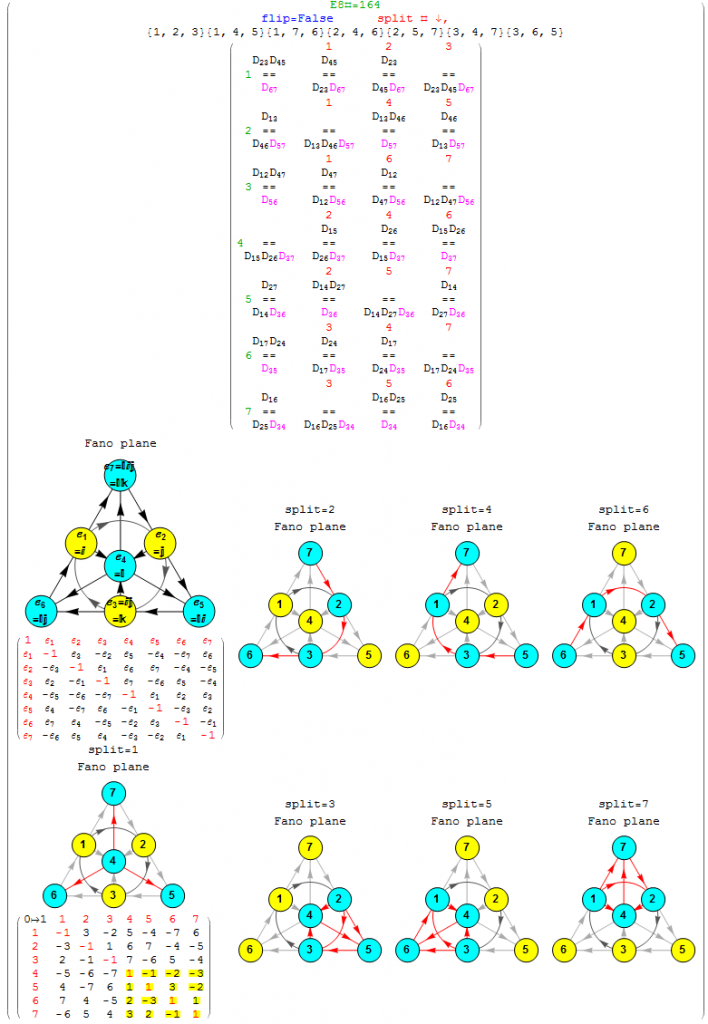Emergent patterns:

1. For each of the 7 non-null derivation triples (the first column of the 7 rows), there are precisely 3 (of the 7) split octonions that don’t share the exact same non-null triple derivation pair as the parent (non-split) octonion (these deviations are shown in column 2 thru 4, along with the associated Fano plane diagrams for the parent and 7 split octonions of E8 #164).
2. Each non-null derivation triple contains 6 of 7 indices, and each row is missing a different index (highlighted in green before the column 1 triple). The sequence of missing element numbers in each row of the given example follows the row number.
3. It is only the equality relationship (signs) of the non-Null triple that change in 3 of the 7 splits, not the D_{x,y} itself.
4. There are always 2 pairs of 2 D_{x,y} which are negative (i.e. with a -1 entry in the NullSpace matrix located above the “==”) that occur across the row.
5. There is always a common positive D_{x,y} (i.e. with a +1 entry in the NullSpace matrix located below the “==”) in each entry of the row (colored magenta). The common positive entries in the 7 rows suggest a “distinguished” non-null indicator for the 14=21-7 G2 automorphism.
6. All octonion multiplication matrices have the first 3 rows of distinguished entries of {6,7},{5,7} and {5,6} in that order (i.e. the last 2 rows of utOct).
7. There are several possible choices for G2 automorphism sets of 14 elements within each of 480 octonions based on the 7 non-Null entries. Interestingly, there are the 4 sets of rows in utOct which sum to 14 elements, specifically rows {{1, 2, 4}, {1, 2, 5, 6}, {1, 2, 4, 6}, {2, 3, 4, 5}}). The distinguished entries of the flipped E8 #164 example below (as in the MathSource post referenced above) suggests a G2 created by rows {1,2,4} of utOct. Although, not all G2 sets must use complete rows as in this example.

This is octonionG2-nonNull, a 480 page (15 MB) which lists each of the 7 non-Null parent octonion derivations (and for each of those, the 3 other split octonion non-null derivations) which are NOT a member of the 14 null derivations for each of 480 octonion multiplication matrices and 7 splits for each (480*8=3840). It includes the Fano plane mnemonics for the parent and 7 split octionions. This is a smaller 240 page (1MB) version of octonionG2-nonNull.pdf without the Fano plane mnemonics.

# Octonion triality testing

I am working on validating some theoretical work on the triality automorphisms of the split octonions. This is a post with preliminary work on that… for those who are interested 🙂

BTW – It requires the here.

An addendum to the original post (below):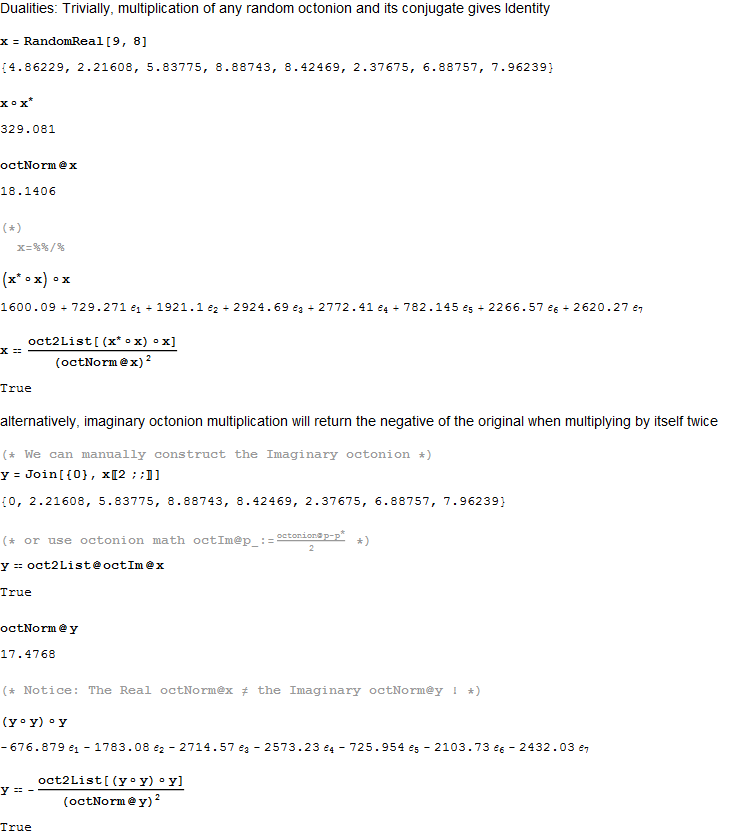[WolframCDF source=”http://theoryofeverything.org/TOE/JGM/octonion triality checks.cdf” width=”900″ height=”8000″ altimage=”http://theoryofeverything.org/TOE/JGM/octonion triality checks.png” altimagewidth=”900″ altimageheight=”8000″]

# Another look at integrating the Pascal Triangle to Clifford Algebra, E8 Lie Algebra/Groups, Octonions and Particle Physics Standard Model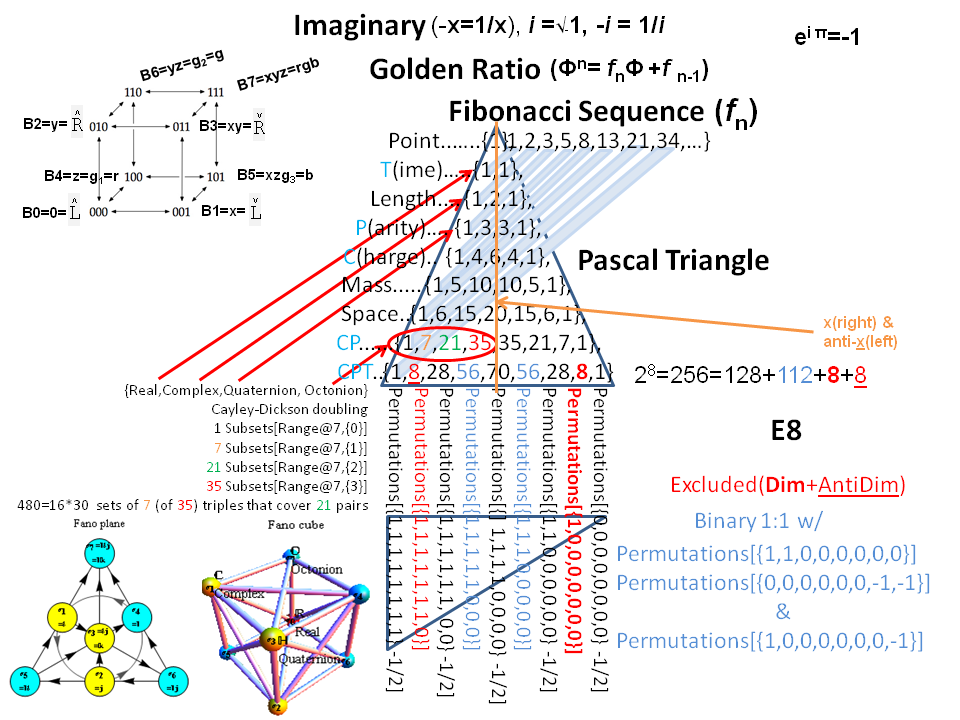Modified Lisi split real even E8 particle assignment quantum bit patterns: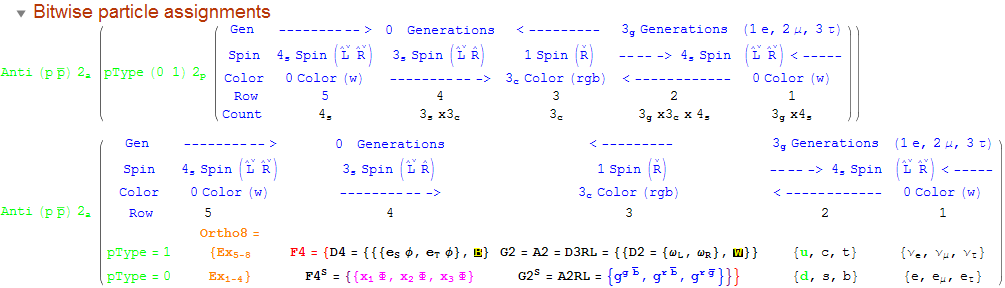Assigning a specific mass, length, time, and charge metrics based on new dimensional relationships and the Planck constant (which defines Higgs mass).The split real even E8 group used has been determined from this simple root matrix (which gives the Cartan matrix upon dot product with a transpose of itself):This Dynkin diagram builds the Cartan matrix and determines the root/weight/height with corresponding Hasse diagrams.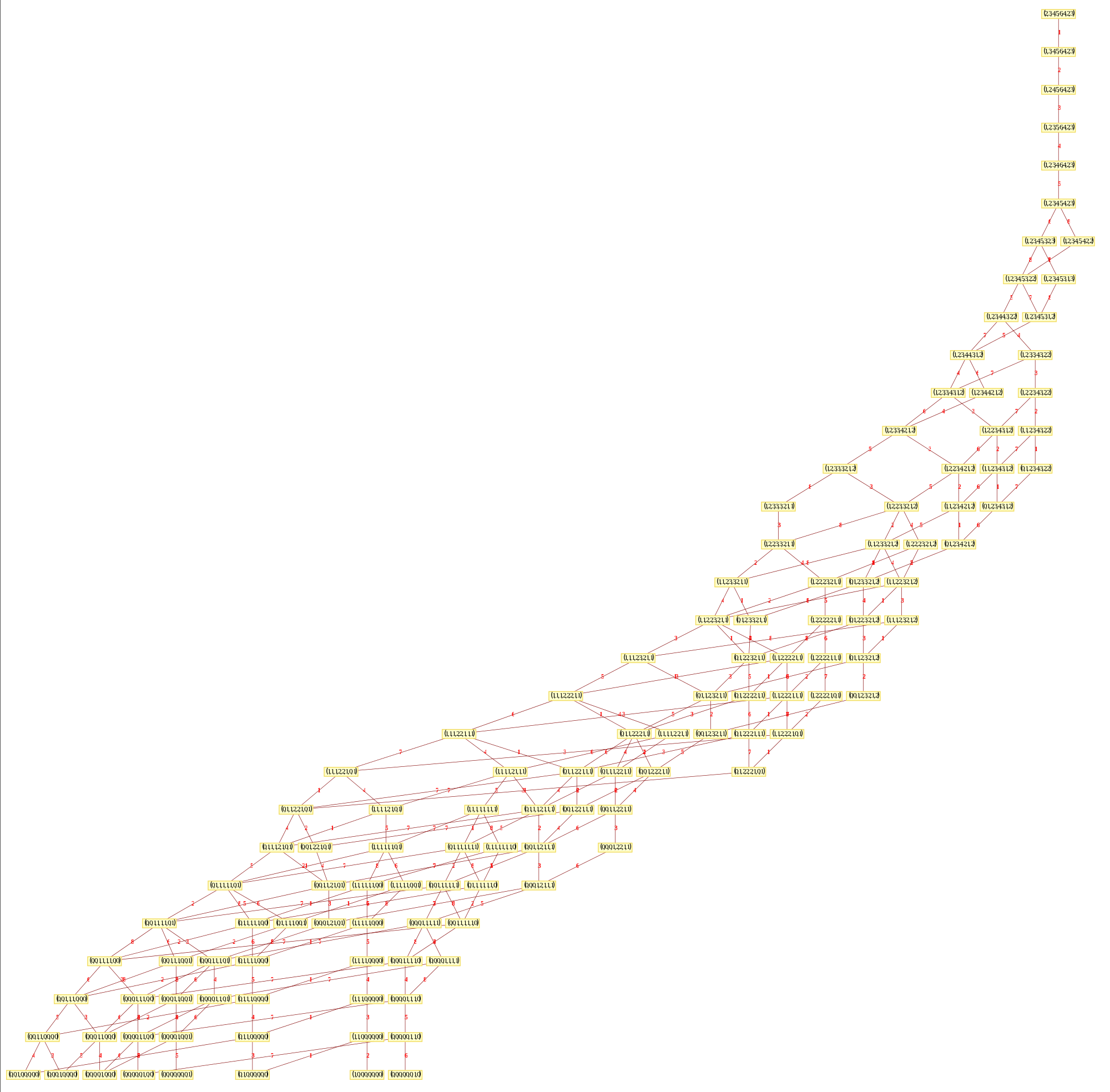# The Comprehensive Split Octonions and their Fano Planes

I am pleased to announce the availability of splitFano.pdf, a 321 page pdf file with the 3840=480*8 split octonion permutations (with Fano planes and multiplication tables). These are organized into “flipped” and “non-flipped” pairs associated with the 240 assigned particles to E8 vertices (sorted by Fano plane index or fPi).

There are 7 sets of split octonions for each of the 480 “parent” octonions (each of which is defined by 30 sets of 7 triads and 16 7 bit “sign masks” which reverse the direction of the triad multiplication). The 7 split octonions are identified by selecting a triad. The complement of {1,2,3,4,5,6,7} and the triad list leaves 4 elements which are the rows/colums corresponding to the negated elements in the multiplication table (highlighted with yellow background). The red arrows in the Fano Plane indicate the potential reversal due to this negation that defines the split octonions. The selected triad nodes are yellow, and the other 4 are cyan (25MB).

These allow for the simplification of Maxwell’s four equations which define electromagnetism (aka.light) into a single equation.

I believe this is the only comprehensive presentation of all 3840 Split Fano Planes with their multiplication tables available.

Below is the first page of the comprehensive split octonion list.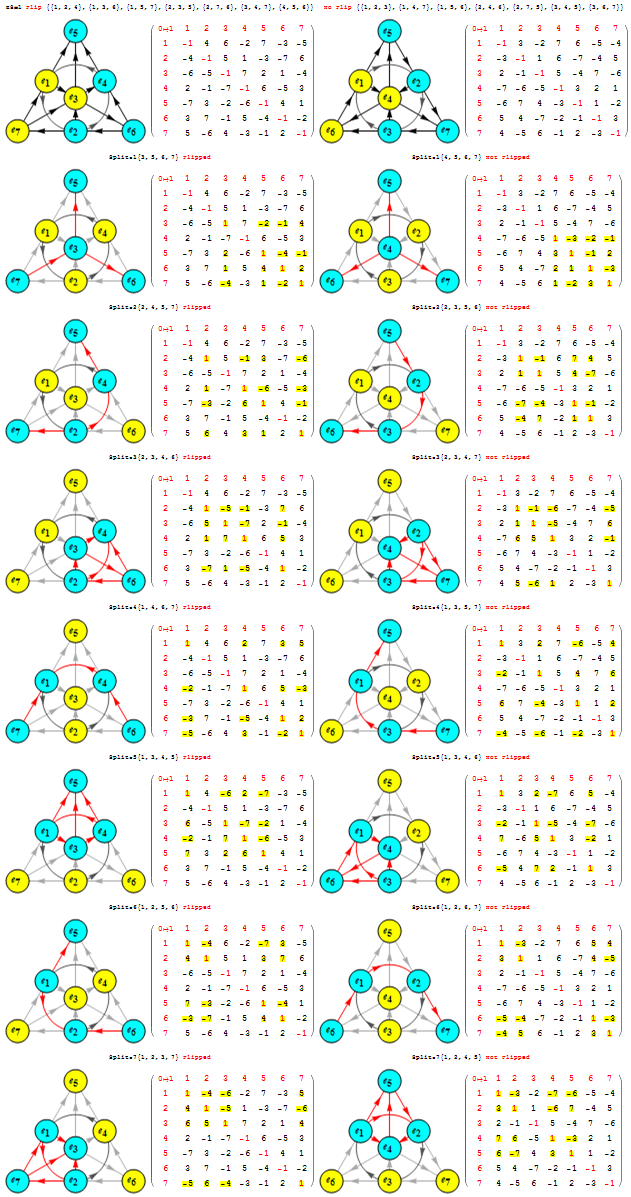# Connecting the Octonion Fano Plane to the Atomic Elements

There are 28 octonion Fano plane triangles that correspond directly to the 28 Trott quartic curve bitangents. These bitangents are directly related to the Legendre functions used in the Shroedinger spherical harmonic electron orbital probability densities. Shown below is a graphic of these overlaid onto the n=5, l=2, m=1 element, which is assigned to gold (Au). When using an algorithm based on the E8 positive algebra root assignments, the “flipped” Fano plane has E8 algebra root number 79 (the atomic number of Au) and split real even group number of 228 (in Clifford/Pascal triangle order). For more information, please see the complete list of integrated Fano indices here with full explanation of the layout here.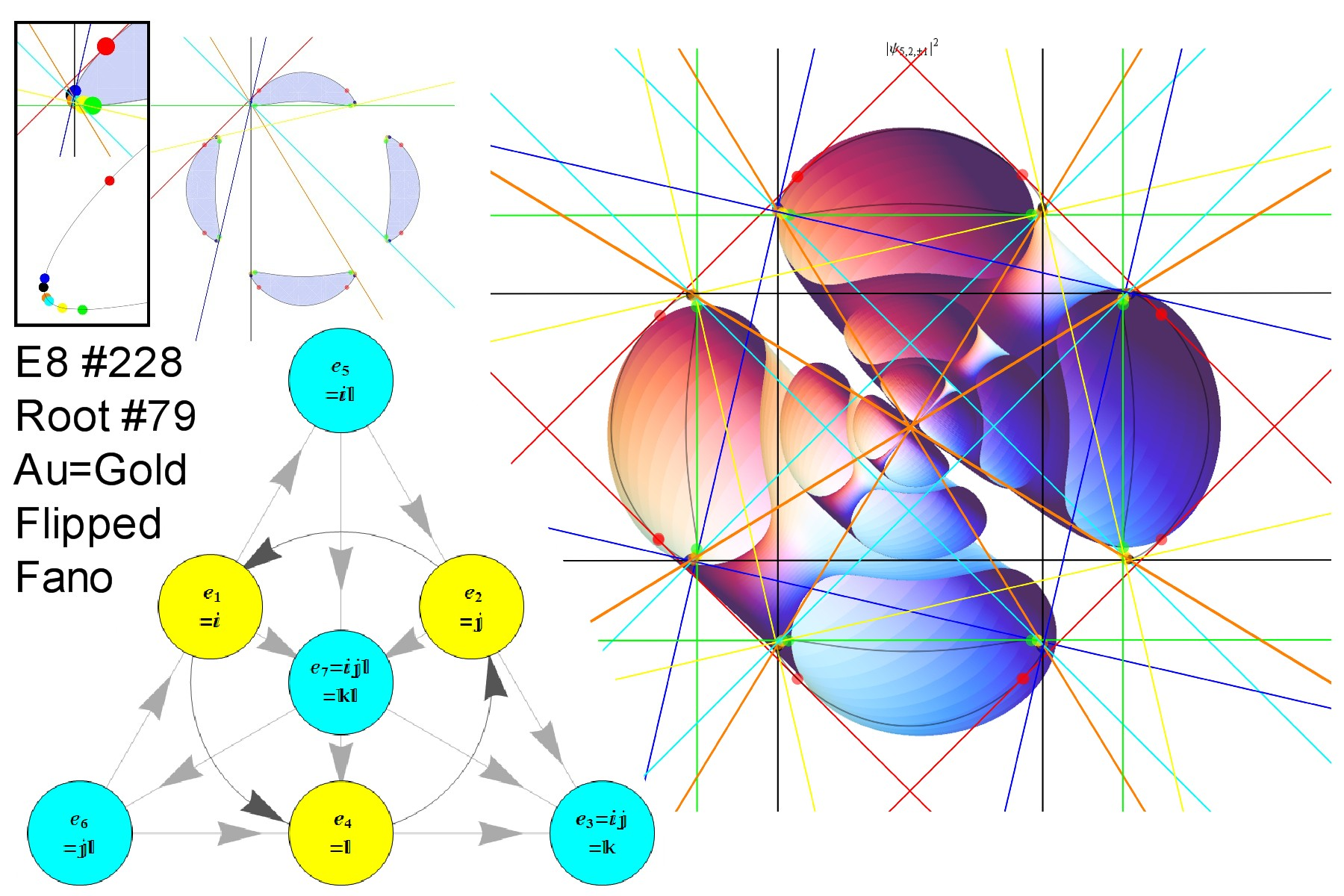# Created a simpler Fano plane and cubic .pdf

A simpler version of Fano.pdf is here FanoOnly.pdf (15MB).